Finding a peak in 1 Dimensional Array

A peak in a 1-D array is simply an element which has left and right elements smaller than it.

1, 3, 4 ,6 ,9, 11, 14, 12, 7, 3, 2

14, clearly is peak here.

A straight forward algorithm would be to parse the array from left to right and keep checking if a[index]>=a[index+1] and a[index]>a[index-1], we have found a peak.

The above algorithm gives me a complexity of O(N).

An improvement over above approach to user divide and conquer approach, like we do in binary search.

1. Go to mid of the array Arr[N/2]
2. Check if it is peak (left and right are smaller)
3. check if left element is larger than mid element, if yes, NewArr= Arr[0..N/2-1]
4. else NewArr=Arr[N/2+1..N]
5. Repeat from 1 with NewArr

Complexity

T(N)=T(N/2)+C //at every step we will divide the array into 2, C is constant time taken in the opertaion
=T(N/4)+C+C
=T(N/8)+C+C+C
=T(N/2^k)+CK
… total number of steps is lg(N)
=T(1)+C*lg(N)

or O(lgN) complexity.

Kruskal’s Algorithm for Minimum spanning tree.

In last post I talked about Prim’s algorithm for minimum spanning tree.

Kruskal is another alorithm to solve same problem.

2. Add edges to a set S in sorted order with increasing order of weight.
3. Take an edge from set S (next minimum weight) and add to graph if (and only if) it is connecting non- cnnected nodes. Igore the edge if vertices are already connected.

So if I try to same problem for this graph.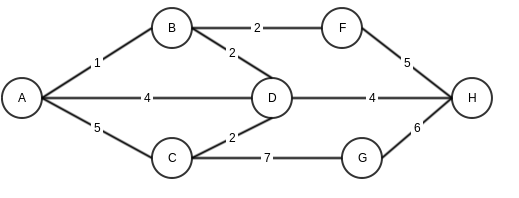These will be the steps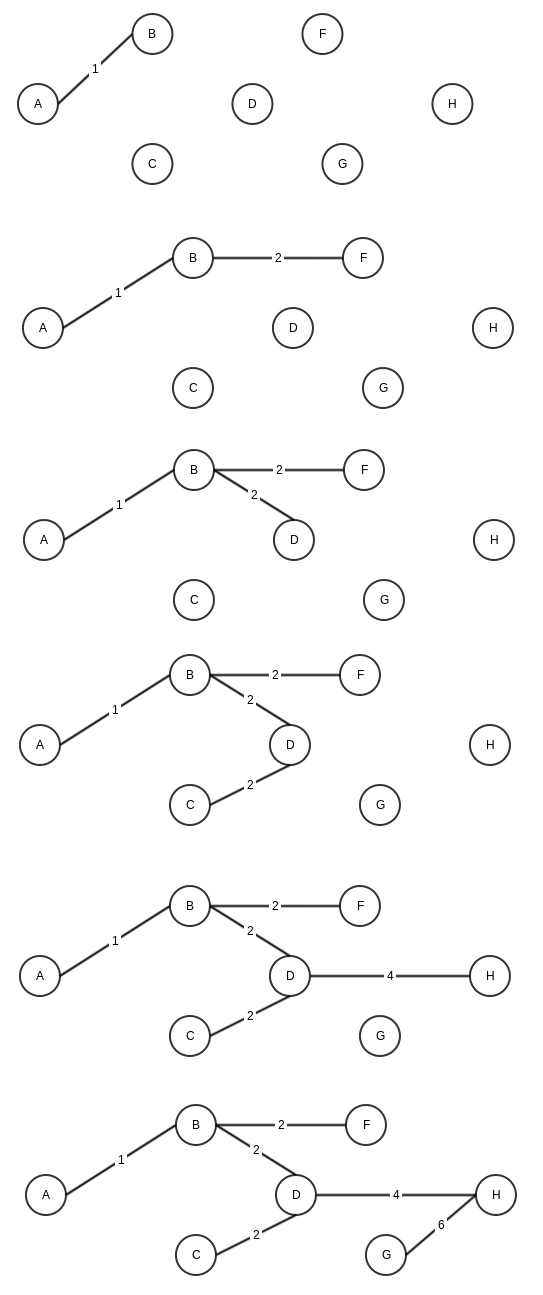Another interesting depiction from wikipedia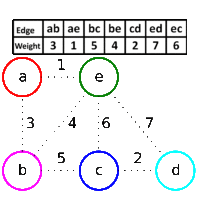Prim’s Algorithm for Minimum spanning tree.

If we look at wikipedia for Prim’s alogorithm, it states

“Prim’s algorithm is a greedy algorithm that finds a minimum spanning tree for a weighted undirected graph”

https://en.wikipedia.org/wiki/Prim%27s_algorithm

Let’s take this line word by word

Greedy algorithm: means this algorithm looks at the best option available at current stage and move stage by stage.

weighted graph: a graph where edges have a weight associated, for example distance as weight in case of a graph showing path between cities.

undirected graph: A graph which does not worry about direction of edges. A edge between vertices A and B would mean it can travel both ways.

Minimum spanning tree: In a graph, a minimum spanning tress is a tree, which has only edges which are required to connect all the nodes and keeping minimum weight.

Now Coming to Prim’s algo, again using wikipedia’s definition

```    1. Initialize a tree with a single vertex, chosen arbitrarily from the graph.
2. Grow the tree by one edge: of the edges that connect the tree to vertices not yet in the tree, find the minimum-weight edge, and transfer it to the tree.
3. Repeat step 2 (until all vertices are in the tree).
```

Let’s say we have a graphHere is step by step execution of Prim’s algorithm (note that for steps where we have multiple same minimum weight options, I am choosing randomly)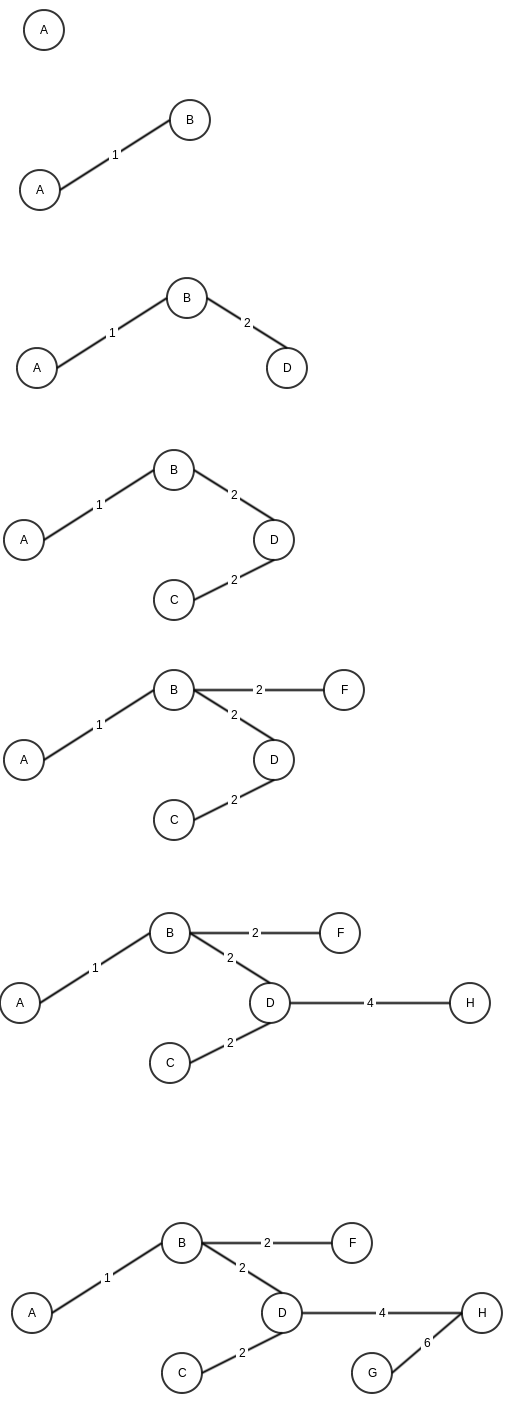Dijkstra’s Algorithm

Sometime back I talked about Graph data structure.

One of the important usage of graph is to find the shortest path from one node to another, for example in travelling salesman problem.

One important algorithm to find shortest path for between two nodes in graph is Dijkstra’s algo.

Here is how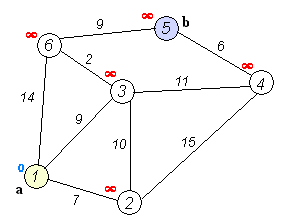Image source wikipedia

1. Mark All node distance as infinity (to mark that node has not been traversed yet)
2. Start from source node, add all other nodes to a set contaning unvisited node
3. Traverse all neighboring nodes from current node N and calculate distance from previous node by adding distance[N] + distance from N to this node (edge weight)
4. When all neighbors are calculated, mark current node as visited.
5. If we have visited the destination node, exit with the distance till destination node.
5. Pull next node from set.
6. Goto 3.

Branch predictor

Whenever in our code a conditional statement is executed, Branch Precitor tries to predict which way the flow will go and executes that even before conditional statement actually returns the output. This is done to stop wastage of time in waiting for conditional statement execution.

From wikipedia
– In computer architecture, a branch predictor is a digital circuit that tries to guess which way a branch (e.g. an if-then-else structure) will go before this is known for sure. The purpose of the branch predictor is to improve the flow in the instruction pipeline. Branch predictors play a critical role in achieving high effective performance in many modern pipelined microprocessor architectures such as x86.

Here is a good example to see what Branch predictor can do

`package Permissions.Permissions;`

``` import java.util.Arrays; import java.util.Random; public class test { public static void main(String[] args) { // Generate data int arraySize = 32768; int data[] = new int[arraySize]; Random rnd = new Random(0); for (int c = 0; c < arraySize; ++c) data[c] = rnd.nextInt() % 256; // !!! With this, the next loop runs faster // Arrays.sort(data); // Test long start = System.nanoTime(); long sum = 0; for (int i = 0; i < 100000; ++i) { // Primary loop for (int c = 0; c < arraySize; ++c) { if (data[c] >= 128) sum += data[c]; } } System.out.println((System.nanoTime() - start) / 1000000000.0); System.out.println("sum = " + sum); } ```

```} ```

Try uncommenting the sort array part and see the difference.

Source- stackoverflow

Longest repeating sub-sequence

Finding longest repeating sub-sequence or substring among a string is classic CS problem. Here is one simple solution where we use approach of finding all the substrings from beginning and sort the resulting array. In sorted array we will find longest common repeating sub-sequence.

Input: banana

Output: ana

//method to return longest subsequence
public static String findLongestSubsequence(String str)
{
String sub=””;

String arr[]=new String[str.length()];
//Firstly lets find all the substrings
for(int i=0;i<str.length();i++)
{
arr[i]=str.substring(i);
}

//as we have the list of all substrings, sort them
Arrays.sort(arr);

//compare strings with next string and find the maximum length common substring
for(int i=0;i<arr.length-1;i++)
{
//compare arr[i], arr[i+1]
int len=(arr[i].length()<arr[i+1].length())?arr[i].length():arr[i+1].length();
String temp=””;

for(int j=0;j<len;j++) { //compare two strings till a point where they are equal if(arr[i].charAt(j)==arr[i+1].charAt(j)) temp=arr[i].substring(0,j+1); else break; } sub=(temp.length()>sub.length())?temp:sub;
}

return sub;
}

There are other ways to find longest repeating sub-sequence. One  important way is to create a m*n matrix with a value showing length of subsequence till that point

b a n a n a
b   0 0 0 0 0 0
a   0 0 0 1 1 1
n   0 0 0 1 2 2
a   0 1 1 1 2 3
n   0 1 2 2 2 3
a   0 1 2 3 3 3

Another sophisticated way is to create a suffix tree. Read more here –http://en.wikipedia.org/wiki/Suffix_tree

Division problem

Problem statement- 3 divides 111 , 13 divides 111111 etc.. find a number having all one’s which is shortest divisible by a given number which has 3 as its last digit.

Logic- The challenge here is that you will soon run out of limit for int or long if you keep on increasing the number. So the idea is to divide in a way which you used to do on paper for large numbers

13)111111(8547
104
——-
71
65
——–
61
54
———-
71
71
————
0

Start with 1 (or 11) and keep adding one, keep a count of 1s added, divide by the required number unless the reminder is 0.

Implementation
```public static void divide(int num) { int division=11; int count=2; while(true) { if(division%num==0) { System.out.println("count:"+count); break; } else { int remindor=division%num; division=remindor*10+1; count++; } } }```

InOrder, PreOrder and PostOrder traversal

I had discussed Breadth first and Depth First traversal algorithms sometime back.

There are 3 important traversing algorithms for tress. http://en.wikipedia.org/wiki/Tree_traversal

InOrder
-Travel Left, Travel Root, Travel Right.

PreOrder
-Root, Left, Right

PostOrder
-Left, Right, Root

Here is recursive implemenation
```public void inOrder(Node root) { if(root.left!=null) inOrder(root.left); System.out.println(root.number); if(root.right!=null) inOrder(root.right); }```
``` public void preOrder(Node root) {```

``` System.out.println(root.number); if(root.left!=null) preOrder(root.left); if(root.right!=null) preOrder(root.right); } ```

```public void postOrder(Node root) { if(root.left!=null) postOrder(root.left); if(root.right!=null) postOrder(root.right); System.out.println(root.number); }```

Depth First Search

In last post I talked about Breadth First Search. An alternative to that, is Depth First Search, used in cases we want to check upto a depth level first rather than breadth.

So let’s look back at the last example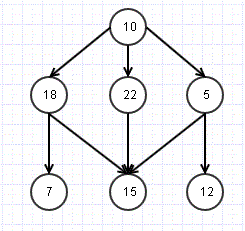This time we would like to parse something like

1. Check Root- 10

2. Check till depth level for one node- 18,7

3. Repeat for other nodes 22,15 and then 5, 12

A straight forward way is to modify the Breadth First Search algorithm to use Stack instead of Queue.

1. Push Root Node R to Stack S
2. Pop s node from S
3 a . If s.val is required value, return Success
3 b. Else Push all Neighbor/ child nodes to stack
4. If Stack is not empty, Repeat from 2
5. If Stack is empty, we have traversed all the nodes and not found the required node. Return failure.

Breadth First search is an effective search mechanism for Graphs and Tree Data structure. As the name suggest, this technique makes sure that all the nodes at same level/ breadth are checked before moving to deeper levels.The above structure is hybrid of tree and graph (Still a tree as there are no cycles). Let us what it means to do a breadth first search here.

Move to level 2 and check nodes 18, 22, 5.

Check the next level- 7, 15, 12 and so on.

Here is algorithm to implement Breadth first search

1. Add root node R to queue- Q

2. Fetch next node N from Q

3 a. If the N.val is required val, return success

3 b. Else Find all Child/ Neighbour nodes which are not traversed already and add to Queue

4. If there are more nodes in Q, Repeat from 2.

5. If Q is empty, we know we have traversed all nodes, return failure as node not found